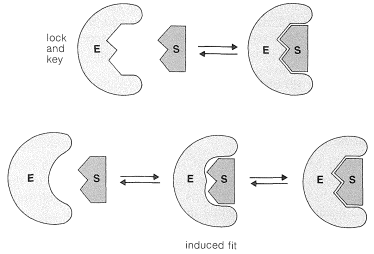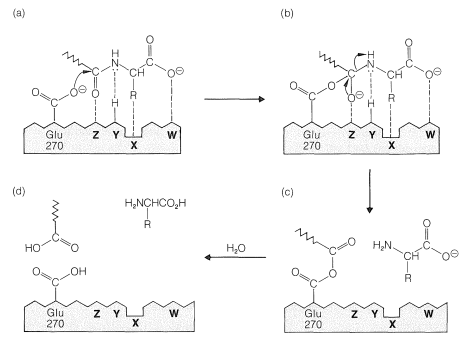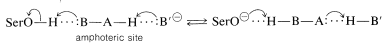# 25.9: Enzymes

$$\newcommand{\vecs}{\overset { \rightharpoonup} {\mathbf{#1}} }$$ $$\newcommand{\vecd}{\overset{-\!-\!\rightharpoonup}{\vphantom{a}\smash {#1}}}$$$$\newcommand{\id}{\mathrm{id}}$$ $$\newcommand{\Span}{\mathrm{span}}$$ $$\newcommand{\kernel}{\mathrm{null}\,}$$ $$\newcommand{\range}{\mathrm{range}\,}$$ $$\newcommand{\RealPart}{\mathrm{Re}}$$ $$\newcommand{\ImaginaryPart}{\mathrm{Im}}$$ $$\newcommand{\Argument}{\mathrm{Arg}}$$ $$\newcommand{\norm}{\| #1 \|}$$ $$\newcommand{\inner}{\langle #1, #2 \rangle}$$ $$\newcommand{\Span}{\mathrm{span}}$$ $$\newcommand{\id}{\mathrm{id}}$$ $$\newcommand{\Span}{\mathrm{span}}$$ $$\newcommand{\kernel}{\mathrm{null}\,}$$ $$\newcommand{\range}{\mathrm{range}\,}$$ $$\newcommand{\RealPart}{\mathrm{Re}}$$ $$\newcommand{\ImaginaryPart}{\mathrm{Im}}$$ $$\newcommand{\Argument}{\mathrm{Arg}}$$ $$\newcommand{\norm}{\| #1 \|}$$ $$\newcommand{\inner}{\langle #1, #2 \rangle}$$ $$\newcommand{\Span}{\mathrm{span}}$$

Virtually all biochemical reactions are catalyzed by proteins called enzymes. The catalytic power and specificity of enzymes is extraordinarily high. The reactions that they catalyze are generally enhanced in rate many orders of magnitude, often as much as $$10^7$$, over the nonenzymatic process. Consequently enzymatic reactions may occur under much milder conditions than comparable laboratory reactions. For example, the simple hydrolysis of an amide proceeds at a practical rate only on heating the amide in either strongly acidic or strongly basic aqueous solution, and even then reaction may not be complete for several hours. In contrast, hydrolysis of amide or peptide bonds catalyzed by typical proteolytic enzymes, such as trypsin, chymotrypsin, or carboxypeptidase A, occurs rapidly as physiological temperatures and physiological pH.$$^7$$ It is one of the remarkable attributes of many enzymes that they catalyze reactions that otherwise would require strongly acidic or basic conditions. Enzymes are strictly catalysts, however, and affect only the rate of reaction, not the position of equilibrium; they lower the energy of the transition state, not the energies of the reactants or products (see Figure 4-4).

Many enzymes appear to be tailor-made for one specific reaction involving only one reactant, which is called the substrate. Others can function more generally with different reactants (substrates). But there is no such thing as a universal enzyme that does all things for all substrates. However, nothing seems to be left to chance; even the equilibration of carbon dioxide with water is achieved with the aid of an enzyme known as carbonic anhydrase.$$^8$$ Clearly, the scope of enzyme chemistry is enormous, yet the structure and function of relatively few enzymes are understood in any detail. We can give here only a brief discussion of the mechanisms of enzyme action - first some general principles then some specific examples.

## Aspects of the Mechanisms of Enzyme Reactions

An enzyme usually catalyzes a single chemical operation at a very specific position, which means that only a small part of the enzyme is intimately involved. The region of the enzyme structure where key reactions occur as the result of association of the substrate with the enzyme is called the active site. The initial association of the enzyme $$\left( \ce{E} \right)$$ and the substrate $$\left( \ce{S} \right)$$ is formation of an enzyme-substrate complex $$\left( \ce{ES} \right)$$:

$\ce{E} + \ce{S} \rightleftharpoons \ce{ES}$

Complexation could occur in many different ways, but for the intimate complexation required for catalysis, the enzyme must have, or must be able to assume, a shape complementary to that of the substrate. Originally, it was believed that the substrate fitted the enzyme somewhat like a key in a lock; this concept has been modified in recent years to the induced-fit theory, whereby the enzyme can adapt to fit the substrate by undergoing conformational changes (Figure 25-18). Alternatively, the substrate may be similarly induced to fit the enzyme. The complementarity is three-dimensional, an important factor in determining the specificity of enzymes to the structure and stereochemical configuration of the substrates.Figure 25-18: Illustration of the lock-and-key concept of enzyme-substrate interaction (top) and of the induced-fit theory, whereby the enzyme molds to the substrate through conformational changes (bottom).

Detailed structures for the active sites of enzymes are difficult to obtain and have been worked out only for a few enzymes that have been studied extensively by both chemical and x-ray methods. Very revealing information has been obtained by x-ray diffraction studies of complexes between the enzyme and nonsubstrates, which are molecules similar to actual substrates and complex with the enzyme at the active site, but do not react further. These substances often inhibit reaction of the normal substrate by associating strongly with the enzyme at the active site and not moving onward to products. The x-ray studies of enzymes complexed with nonsubstrates show that the active site generally is a cleft or cavity in the folded structure of the enzyme that is largely hydrophobic in character. The enzyme-substrate complex can be inferred to be held together largely by van der Waals attractive forces between like groups (Section 12-3C), hydrogen-bonding, and by electrostatic attraction between ionic or polar groups. To achieve a stereospecific catalyzed reaction, there must be at least three points of such interactions to align properly the substrate within the cavity of the enzyme.

The reaction of the $$\ce{ES}$$ complex may convert the substrate to product $$\left( \ce{P} \right)$$ directly, and simultaneously free the enzyme $$\left( \ce{E} \right)$$ to react with more of the substrate:

$\ce{ES} \rightarrow \ce{P} + \ce{E}$

However, the reaction between enzyme and substrate often is much more complex. In many cases, the substrate becomes covalently bound to the enzyme. Then, in a subsequent step, or steps, the enzyme-bound substrate $$\left( \ce{ES'} \right)$$ reacts to give products and regenerate the active enzyme $$\left( \ce{E} \right)$$:

$\ce{ES} \rightarrow \ce{ES'} \rightarrow \ce{P} + \ce{E}$

## Carboxypeptidase A

The considerable detail to which we now can understand enzyme catalysis is well illustrated by what is known about the action of carboxypeptidase A. This enzyme (Section 25-7B and Table 25-3) is one of the digestive enzymes of the pancreas that specifically hydrolyze peptide bonds at the $$\ce{C}$$-terminal end. Both the amino-acid sequence and the three-dimensional structure of carboxypeptidase A are known. The enzyme is a single chain of 307 amino-acid residues. The chain has regions where it is associated as an $$\alpha$$ helix and others where it is associated as a $$\beta$$-pleated sheet. The prosthetic group is a zinc ion bound to three specific amino acids and one water molecule near the surface of the molecule. The amino acids bound ot zinc are His 69, His 196, and Glu 72; the numbering refers to the position of the amino acid along the chain, with the amino acid at the $$\ce{N}$$-terminus being number 1. The zinc ion is essential for the activity of the enzyme and is implicated, therefore, as part of the active site.

X-ray studies$$^9$$ of carboxypeptidase complexed with glycyltyrosine (with which it reacts only slowly) provide a detailed description of the active site, which is shown schematically in Figure 25-19a and is explained below.Figure 25-19: Steps in a possible mechanism of carboxypeptidase action. (a) The substrate is shown complexed to the enzyme surface through $$\ce{X}$$, $$\ce{Y}$$, $$\ce{Z}$$, and $$\ce{W}$$; $$\ce{X}$$ is a nonpolar pocket; $$\ce{Y}$$ is a hydrogen bond, possibly from $$\ce{OH}$$ of Tyr 248; $$\ce{Z}$$ is the prosthetic group, $$\ce{Zn}$$; and $$\ce{W}$$ is an ionic interaction with $$\ce{=} \overset{\oplus}{\ce{N}} \ce{H_2}$$ of Arg 145. The $$\ce{C}$$-terminal amide bond of the substrate is held close to the catalytic site, which is the carboxyl of Glu 270. (b) A tetrahedral intermediate could be formed by attack of Glu 270 carboxylate anion at the amide carbonyl of the substrate. (c) Cleavage of the tetrahedral intermediate of (b) releases the $$\ce{C}$$-terminal amino acid and forms an acyl-enzyme intermediate. (d) The residue of the substrate chain is released from the enzyme by hydrolysis of the acyl-enzyme intermediate. These drawings are deficient in that they try to reproduce a three-dimensional situation in two dimensions. The third dimension is especially important in understanding the stereospecificity of the enzyme.

1. The tyrosine carboxylate group of the substrate is associated by electrostatic attraction with the positively charged side chain of arginine 145 $$\left( \ce{W} \right)$$:2. The tyrosine side chain of the substrate associates with a nonpolar pocket in the enzyme $$\left( \ce{X} \right)$$.
3. Hydrogen bonding possibly occurs between the substrate tyrosine amide unshared pair and the side-chain $$\ce{HO}$$ groups of the enzyme tyrosine 248 $$\left( \ce{Y} \right)$$.
4. The glycyl carbonyl oxygen in the substrate probably is coordinated with the zinc ion $$\left( \ce{Z} \right)$$, displacing the water molecule coordinated to the zinc in the uncomplexed enzyme.
5. A side-chain carboxylate anion of glutamic acid 270 is so situated with respect to the reaction center that it could well function as a nucleophile by attacking the glycine carbonyl carbon.

The arrangement of the enzyme-substrate complex suggests a plausible reaction mechanism analogous to nonenzymatic mechanisms of amide hydrolysis (Section 24-4). The carboxyl group of Glu 270 can add to the amide carbonyl to form a tetrahedral intermediate that then rapidly dissociates to release the terminal amino acid, leaving the rest of the substrate bound to the enzyme as a mixed anhydride which can be symbolized as $$\ce{E(CO)OCOR_1}$$. Reaction of the acyl-enzyme intermediate with water will release the peptide, minus the terminal amino acid, and regenerates the enzyme.

This postulated sequence of events may leave you wondering why the enzyme speeds up the hydrolysis, especially because the sequence proceeds through an energetically unfavorable reaction, the formation of a carboxylic anhydride from an amide and a carboxylic acid:Figure 25-20 is shown with a higher-energy transition state than with water, as would be expected, because $$\ce{CH_3CO_2H}$$ is a poorer nucleophile than water.Figure 25-20: Possible energy profiles for different pathways for hydrolysis of a simple amide. The dashed line represents an enzyme-catalyzed formation of $$\ce{R(CO)OCOCH_3}$$, which is here hypothesized to have a lower-energy transition state than the reaction of the anhydride with water (see text).

How, then, can we possibly expect that the enzyme could make the anhydride route be faster than the simple water route? The answer is the way in which interactions in the enzyme-substrate complex can stabilize the transition state for the anhydride-forming reaction. Thus for carboxypeptidase the zinc can act as a strong electrophile to facilitate attack on the amide carbonyl. Hydrogen bonding of the amide nitrogen to Tyr 248 both will facilitate attack on the carbonyl group and assist in the breaking of the $$\ce{C-N}$$ bond. Furthermore, the nonpolar environment of the alkyl side-chains of the enzyme will increase the nucleophilicity of the $$\ce{-CO_2^-}$$ group that forms the anhydride (see Section 8-7F). Inspection of Figure 25-20 shows qualitatively that if the energy of the transition state for formation of the anhydride is lowered greatly, the overall rate will be determined by the rate of hydrolysis of the anhydride! In this circumstance (assuming the anhydride hydrolysis is uncatalyzed), the efficiency of the enzyme in breaking the peptide bond is as great as it can be, at least by this particular pathway of peptide hydrolysis. Such efficiencies have been established for other enzymes.

## Participation of Serine in Enzyme Action

Mechanisms similar to the one described for carboxypeptidase appear to operate in the hydrolysis of amide and ester bonds catalyzed by a number of proteinases and esterases. The substrate, here generalized as $$\ce{RCOX}$$, acts to acylate the enzyme, which subsequently reacts with water to give the observed products:In the acylation step a nucleophilic group on one of the amino-acid side chains at the active site behaves as the nucleophile. As we have seen in Section 25-9B, the nucleophile of carboxypeptidase is the free carboxyl group of glutamic acid 270. In several other enzymes (chymotrypsin, subtilisin, trypsin, elastase, thrombin, acetylcholinesterase), it is the hydroxyl group of a serine residue:This raises another question. Why is the serine hydroxyl an effective nucleophile when water and other hydroxylic compounds clearly are not similarly effective? Apparently, the nucleophilicity of the serine $$\ce{-CH_2OH}$$ is enhanced by acid-base catalysis involving proton transfers between acidic and basic side-chain functions in the vicinity of the active site. The serine is believed to transfer its $$\ce{OH}$$ proton to an amphoteric$$^{10}$$ site $$\ce{B-A-H}$$ on the enzyme at the same instant that the proton of $$\ce{B-A-H}$$ is transferred to another base $$\ce{B'}^\ominus$$ (Equation 25-8). These proton transfers are, of course, reversible:$$\tag{25-8}$$

Loss of its proton makes the serine hydroxyl oxygen a much more powerful nucleophile, and even though the equilibrium of Equation 25-8 must lie far to the left at physiological pH, it can increase greatly the reactivity of the serine hydroxyl.

In chymotrypsin and subtilisin, this charge-relay network system, as it is called, is made up of a specific aspartic acid residue, acting as $$\ce{B'}^\ominus$$, and a specific histidine residue (acting as the amphoteric $$\ce{B-A-H}$$):$$^7$$The slowness with which amide bonds are hydrolyzed in the presence of either strong acids or strong bases, and their susceptibility to hydrolysis under the influence of enzymes, clearly is a key advantage in the biological functioning of peptides. Amide hydrolysis in neutral solution has a favorable, but not large, equilibrium constant. Therefore it does not take a great deal of biochemical energy either to form or to hydrolyze peptide bonds. The resistance to ordinary hydrolysis provides needed stability for proteins, and yet when it is necessary to break down the peptide bonds of proteins, as in digestion, this can be done smoothly and efficiently with the aid of the proteolytic enzymes.

$$^8$$Many enzymes are named by adding the suffix -ase to a word, or words, descriptive of the type of enzymatic activity. Thus, esterases hydrolyze esters, proteinases hydrolyze proteins, reductases achieve reductions, and synthetases achieve synthesis of polypeptide chains, nucleic acid chains, and other molecules.

$$^9$$W. N. Lipscomb, Accounts of Chemical Research 3, 81 (1970); E. T. Kaiser and B. L. Kaiser, ibid. 5, 219 (1972). Lipscomb received the 1976 Nobel Prize in chemistry for structural work on boranes.

$$^{10}$$Amphoteric means that a substance can act either as an acid or as a base.